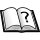Normal view

# Numerical analysis / Richard L. Burden, J. Douglas Faires.

Material type:TextPublication details: Delhi : Cengage Learning, c2011.Edition: 9th edDescription: xiv, 872 p. : col. ill. 27 cmISBN:
• 9788131516546
Subject(s): DDC classification:
• BUR 518
Contents:
Mathematical preliminaries and error analysis -- Solutions of equations in one variable -- Interpolation and polynomial approximation -- Numerical differentiation and integration -- Initial-value problems for ordinary differential equations --Direct methods for solving linear systems -- Iterative techniques in matrix algebra -- Approximation theory -- Approximating eigenvalues -- Numerical solutions of nonlinear systems of equations -- Boundary-value problems for ordinary differential equations -- Numerical solutions to partial differential equations.
Tags from this library: No tags from this library for this title.
Star ratingsAverage rating: 0.0 (0 votes)
Holdings
Item type Current library Collection Call number Copy number Status Notes Date due Barcode Item holdsReference IIT Goa Central Library Reference 518/BUR (Browse shelf(Opens below)) 1 Reference TTPP/103/2023-24||14-07-2023||20%|| 4181Books IIT Goa Central Library 518/BUR (Browse shelf(Opens below)) 2 Available TTPP/152/2023-24||21-08-2023||20.00% 4221
Total holds: 0

Includes bibliographical references (p. 763-772) and index.

Mathematical preliminaries and error analysis -- Solutions of equations in one variable -- Interpolation and polynomial approximation -- Numerical differentiation and integration -- Initial-value problems for ordinary differential equations --Direct methods for solving linear systems -- Iterative techniques in matrix algebra -- Approximation theory -- Approximating eigenvalues -- Numerical solutions of nonlinear systems of equations -- Boundary-value problems for ordinary differential equations -- Numerical solutions to partial differential equations.

Share